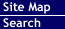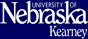Offered by Department of Mathematics and Statistics
College of Natural and Social Sciences

### STAT Courses

STAT 235 - Statistical Techniques for Research I - 3 hours
Prereq: MATH 101
Placement: ACT ³ 20** and 2 years of high school algebra
An introduction to statistics for educational and sociological research. The course will include descriptive statistics, normal distribution and an introduction to correlation and hypothesis testing.
STAT 241 - Elementary Statistics - 3 hours
Prereq: MATH 101
Placement: ACT ³ 20** and 2 years of high school algebra
An introduction to statistical concepts and methods. Not open to department majors.
STAT 345 - Applied Statistics I - 3 hours
Prereq: MATH 115* or MATH 123*
Descriptive statistics; statistical inference using the binomial, normal, F and Chi Square distributions; and analysis of variance topics. Recommended for departmental majors as the beginning applied statistics course.
STAT 399 - Internship - 1-4 hours
Prereq: MATH 115* or MATH 123*
On the job experience designed to complement the major. Internships are available only in selected areas. Consult with departmental advisor. (Credit/No Credit)
STAT 437/837P - Computer Analysis of Statistical Data - 3 hours
Prereq: One computer course; STAT 241*; STAT 345*
The analysis of data taken from the social sciences. The computer will be a tool for the course.
STAT 441/841P - Probability and Statistics - 3 hours
Prereq: MATH 260*
The mathematical development of discrete and continuous probability distributions including multivariate distributions, moments and moment generating functions, the special discrete and continuous probability distributions, the normal distribution, sampling distributions, and hypothesis testing.
STAT 442/842P - Mathematical Statistics - 3 hours
Prereq: STAT 345*, STAT 441*
A continuation of 441/841P. The further mathematical development of special probability densities, functions of random variables, sampling distributions, decision theory, point and interval estimators, hypotheses testing, and covariance.
STAT 495/895P - Independent Study in Statistics - 1-3 hours
Prereq: permission
An individual investigation by the student of topics not included in the normal statistics offerings.

24 Oct 2006